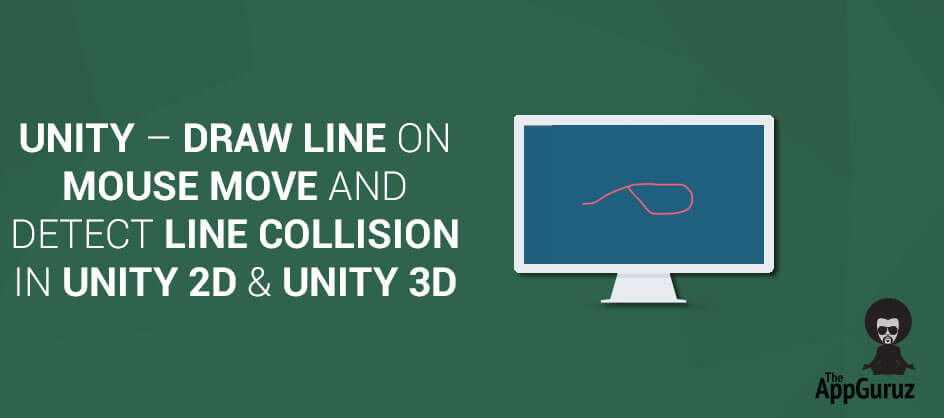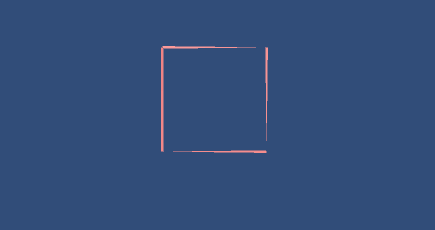## Objective

The Main objective of this Code sample is to explain how to Draw Line on mouse move and Detect Line Collision in Unity3d.

In unity, Line Renderer component gives the facility to draw a line as per our requirement. Before going into Implementation, I would like to throw some light on the unity terms which we are going to use here. If You are already familiar with it, you can skip it and move on to the implementation.

## Step 1What is Line Renderer?

The Line Renderer is used to draw free-floating lines in 3D space or 2D Space. We can draw any kind of Curved Lines (For example, Bézier curve ) as well using Line Renderer and playing around with its components (i.e properties).

A Line Renderer component draws a straight line between points. It takes array of points as vector3 and draws a single straight line between points in array in sequential order. Thus, a single line renderer component can be used to draw anything from simple straight line to any complex shape.

A line drawn by Line Renderer is always continuous. If we want to draw separate line or shape, we have to take multiple GameObjects, each with its own line renderer component attached to it. The Line Renderer does not render one pixel thin lines. It renders billboard lines that have width and can be textured.

### 1.1Some useful properties of Line Renderer

>> We can extend the line by increasing the size as per requirement.

Properties:

 Material Materials is the list of all materials on this object and first material is used to Render the lines. Position Array of vector3 points. Size Number of points in this line.

### Note

To get smother curves always have larger number of vertexes

### 1.2Parameters of Line Render

Parameters:

 StartWidth Width at first line position. EndWidth Width at last line position. StartColor Color at first line position. EndColor Color at last line position. UseWorldSpace If enabled, object’s position is ignored and line rendered at world position.

### Note

Here the other position colors and width are calculated corresponding to the starting and end position properties as given above.

### 1.3Functions of Line Renderer used in the code

``public void SetColors(Color start, Color end);``

Used for setting the start color and end color properties of line Renderer.

``public void SetPosition(int index, Vector3 position);``

Set the position of the vertex in the line.

``public void SetVertexCount(int count);``

Set the number of line segments.

``public void SetWidth(float start, float end);``

Set the line width at the start and at the end.

### 1.4Simple LineRenderer Example

Let us understand LineRenderer by taking a simple example first. Let us Draw a simple Rectangle using LineRenderer.

#### 1.4.1Scene Setup

• Take an empty GameObject.
• Apply Draw Rectangle Script as given below.
• Add LineRenderer Component to it.

### Challenge

• Add Multiple Materials and check its Effect.

Keep to start width and end width to 0.1f.#### 1.4.2Code DrawRectangle.cs

``````public class DrawRectangle : MonoBehaviour
{
LineRenderer line;

void Start ()
{
line = transform.GetComponent<LineRenderer> ();
line.SetVertexCount (5);
line.SetPosition (0, new Vector3 (-1, 1, 0));
line.SetPosition (1, new Vector3 (1, 1, 0));
line.SetPosition (2, new Vector3 (1, -1, 0));
line.SetPosition (3, new Vector3 (-1, -1, 0));
line.SetPosition (4, new Vector3 (-1, 1, 0));
}
}``````

### Challenge

• Can you Draw A Circle using LineRenderer?

## Step 2Scene Setup for Collision Detection of LineRenderer in Unity 3D

Take An Empty GameObject in the Scene.

Attach the DrawLine.cs script to the GameObject

Save the Scene.

## Step 3Understanding The Script

Here we are trying to Detect Collision if a line intersects with itself at any point of time while drawing.

This collision Detection can be achieved by using this given script.

Output:Script: DrawLine.cs

``````using UnityEngine;
using System.Collections;
using System.Collections.Generic;

public class DrawLine : MonoBehaviour
{
private LineRenderer line;
private bool isMousePressed;
public List<Vector3> pointsList;
private Vector3 mousePos;

// Structure for line points
struct myLine
{
public Vector3 StartPoint;
public Vector3 EndPoint;
};
//	-----------------------------------
void Awake ()
{
// Create line renderer component and set its property
line.SetVertexCount (0);
line.SetWidth (0.1f, 0.1f);
line.SetColors (Color.green, Color.green);
line.useWorldSpace = true;
isMousePressed = false;
pointsList = new List<Vector3> ();
//		renderer.material.SetTextureOffset(
}
//	-----------------------------------
void Update ()
{
// If mouse button down, remove old line and set its color to green
if (Input.GetMouseButtonDown (0)) {
isMousePressed = true;
line.SetVertexCount (0);
pointsList.RemoveRange (0, pointsList.Count);
line.SetColors (Color.green, Color.green);
}

if (Input.GetMouseButtonUp (0)) {
isMousePressed = false;
}
// Drawing line when mouse is moving(presses)
if (isMousePressed) {
mousePos = Camera.main.ScreenToWorldPoint (Input.mousePosition);
mousePos.z = 0;
if (!pointsList.Contains (mousePos)) {
line.SetVertexCount (pointsList.Count);
line.SetPosition (pointsList.Count - 1, (Vector3)pointsList [pointsList.Count - 1]);
if (isLineCollide ()) {
isMousePressed = false;
line.SetColors (Color.red, Color.red);
}
}
}
}
//	-----------------------------------
//  Following method checks is currentLine(line drawn by last two points) collided with line
//	-----------------------------------
private bool isLineCollide ()
{
if (pointsList.Count < 2)
return false;
int TotalLines = pointsList.Count - 1;
myLine[] lines = new myLine[TotalLines];
if (TotalLines > 1) {
for (int i=0; i<TotalLines; i++) {
lines [i].StartPoint = (Vector3)pointsList [i];
lines [i].EndPoint = (Vector3)pointsList [i + 1];
}
}
for (int i=0; i<TotalLines-1; i++) {
myLine currentLine;
currentLine.StartPoint = (Vector3)pointsList [pointsList.Count - 2];
currentLine.EndPoint = (Vector3)pointsList [pointsList.Count - 1];
if (isLinesIntersect (lines [i], currentLine))
return true;
}
return false;
}
//	-----------------------------------
//	Following method checks whether given two points are same or not
//	-----------------------------------
private bool checkPoints (Vector3 pointA, Vector3 pointB)
{
return (pointA.x == pointB.x && pointA.y == pointB.y);
}
//	-----------------------------------
//	Following method checks whether given two line intersect or not
//	-----------------------------------
private bool isLinesIntersect (myLine L1, myLine L2)
{
if (checkPoints (L1.StartPoint, L2.StartPoint) ||
checkPoints (L1.StartPoint, L2.EndPoint) ||
checkPoints (L1.EndPoint, L2.StartPoint) ||
checkPoints (L1.EndPoint, L2.EndPoint))
return false;

return((Mathf.Max (L1.StartPoint.x, L1.EndPoint.x) >= Mathf.Min (L2.StartPoint.x, L2.EndPoint.x)) &&
(Mathf.Max (L2.StartPoint.x, L2.EndPoint.x) >= Mathf.Min (L1.StartPoint.x, L1.EndPoint.x)) &&
(Mathf.Max (L1.StartPoint.y, L1.EndPoint.y) >= Mathf.Min (L2.StartPoint.y, L2.EndPoint.y)) &&
(Mathf.Max (L2.StartPoint.y, L2.EndPoint.y) >= Mathf.Min (L1.StartPoint.y, L1.EndPoint.y))
);
}
}``````

### 3.1Depth Understanding of the Code

``````struct myLine
{
public Vector3 StartPoint;
public Vector3 EndPoint;
};``````

Here we have declared an structure to keep track of each and everyline segments in the LineRenderer.

In Awake() we initializing all the components of a LineRender which we have already discussed above.

In Update() we are checking if the mouse click is down we start storing the points in the renderer as well as in a temp list known as pointList. using the statement.

Now If Mouse click is pressed we check if line is colliding with any part of that line if call Method isLineCollide(), which is called on each Update() call.

In isLineCollide() we seperate it in a struct type and than check to see if any of the line segments is colliding with any other line segment stored in the LineRender by Calling isLinesIntersect().

We check each and every Point of line Segements by calling checkPoints() for every for every two line segments , which will return false if intersection is not found, and will return true if intersection is found.

## Challenges

1. Can Collision be detected without calculating for intersection for each and every point?
2. Is it Effective way for detecting intersection?
3. Can collision of two different LineRenders be checked using this technique?
4. Can collisions between a LineRenderer and Other GameObjects be detected?
5. Can you Check Collision Detection using Co-routine, instead from the continuous Update() Method?

## Conclusion

This is one of the effective way of detecting collision in line segments, it is a bit overkill, but if the game is more focused on the Lines itself m this can be easily used.

If you have got any query related How to Draw Line on mouse move and detect line collision in Unity2d and Unity3d then comment them below we will try to solve them out.

Got an Idea of Game Development? What are you still waiting for? Contact us now and see the Idea live soon. Our company has been named as one of the best Game Development Company in India.I am professional Game Developer, developing games in cocos2d(for iOS) and unity(for all platforms). Games are my passion and I aim to create addictive, high quality games. I have been working in this field since 1 year.